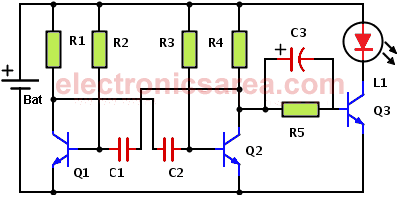1.5 v Joule Thief Circuit

This 1.5 V Joule Thief circuit allows you to drive a LED. The circuit do not use a toroid, so it can not handle a blue or white high intensity LEDs.

Remember that normally the red LEDs light up, barely acceptable way, when there is a minimum of 1.5 volts at its terminals.

Knowing that the voltage drop between the collector and the emitter of transistor Q3 (in series with the LED) is about 0.2 volts, the voltage on the LED will be 1.3 volts and the lighting would be poor. If you want to use LEDs of other colors such as green and yellow, the lighting will also be inefficient, because the voltage needed to illuminate these LEDs is greater.To make the LED lights with good light intensity, we have to increase the voltage applied across its terminals. To achieve this goal we use an electrolytic capacitor of 1uF, which will “save” an additional voltage. This voltage is in series with the 1.5V battery allowing the LED to emit a light of greater intensity.

How the 1.5 v Joule Thief works?

The left side of the 1.5 v Joule Thief Circuit diagram, with transistors Q1, Q2 and associated elements (R1, R2, R3, R4, C1 and C2 ), is an astable multivibrator. The multivibrator output is taken from the collector of transistor Q2.

• When transistor Q2 is off, transistor Q3 goes into saturation, so that a current flows through the resistors R4 and R5. The capacitor C3 will be charged to a voltage of about 1 volt.
• When the transistor Q2 goes into saturation, the collector of this transistor is almost 0 volts. So the left terminal of the capacitor (with the “+” sign) is also at 0 volts.

As we said before, the capacitor is already charged and between its terminals there are approximately 1 volt, then at the cathode of the LED we have -1 volt.

From the diagram of the 1.5 v Joule Thief Circuit  we can see that the anode of the LED is connected to +1.5 volts and its cathode is connected to -1 volt. With this we get a voltage: Vd = 1.5V – (- 1V) = 2.5 volts on LED terminals. This cycle, with a frequency set by the astable multivibrator is repeated indefinitely.

With 2.5 volts is not possible to light up a white or blue high intensity LED, because the supply voltage needed is about 3.7 volts.

List of components for the 1.5 v Joule Thief Circuit

• 3  PNP 2N2222A transistors (Q1, Q2, Q3)
• 1  2.2K resistor (R1)
• 2  4.7K resistors (R2, R3)
• 1  100K resistor (R4)
• 1  1K resistor (R5)
• 2  10 nF capacitor (C1, C2)
• 1  electrolytic capacitor 1uF (C3)
• 1  red, green or yellow LED (L1)
• 1  1.5 volt battery (Bat)

•
•
•
•
•
•
•
•
•
•
•
•
•
•
•
•
•
•
Tagged with: